## A -9.00 μC point charge and +7.00 μC point charge are placed along the x-axis at x = 0.000 m and x = 0.4 m, respectively. Where must a third

Question

A -9.00 μC point charge and +7.00 μC point charge are placed along the x-axis at x = 0.000 m and x = 0.4 m, respectively. Where must a third charge be placed along the x-axis (relative to the origin) so that it does not experience any net electric force due to the other two charges? The position on the x-axis is in meters and use three significant digits.

in progress 0
2 months 2021-07-24T09:29:49+00:00 1 Answers 7 views 0

x₃ = 0.397 m

Explanation:

For this exercise we use the vector sum of the forces, where the force is electric given by the Coulomb equation

F =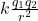We also use that charges of the same sign repel and charges of a different sign attract.

In this case the force between load 1 and 3 has one direction and the force between 2 and 3 has the opposite direction, in the exercise they ask that the force on load 3 be zero

∑ F = F₁₃ – F₂₃ = 0

F₁₃ = F₂₃

we write the expressions of the forces, the distances are

r₁₃ = (x₁-x₃) ²

r₂₃ = (x₂ – x₃) ²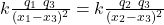x₁ = 0 m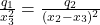q₁ (x₂ – x₃) ² = q₂ x₃²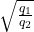(x₂ -x₃) = x₃

we substitute the values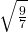(0.4 -x₃) = x₃

x₃ (1 +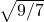) =0.4

x₃ (1.13389) = 0.453557

x₃ = 0.453557 / 1.13389

x₃ = 0.397 m